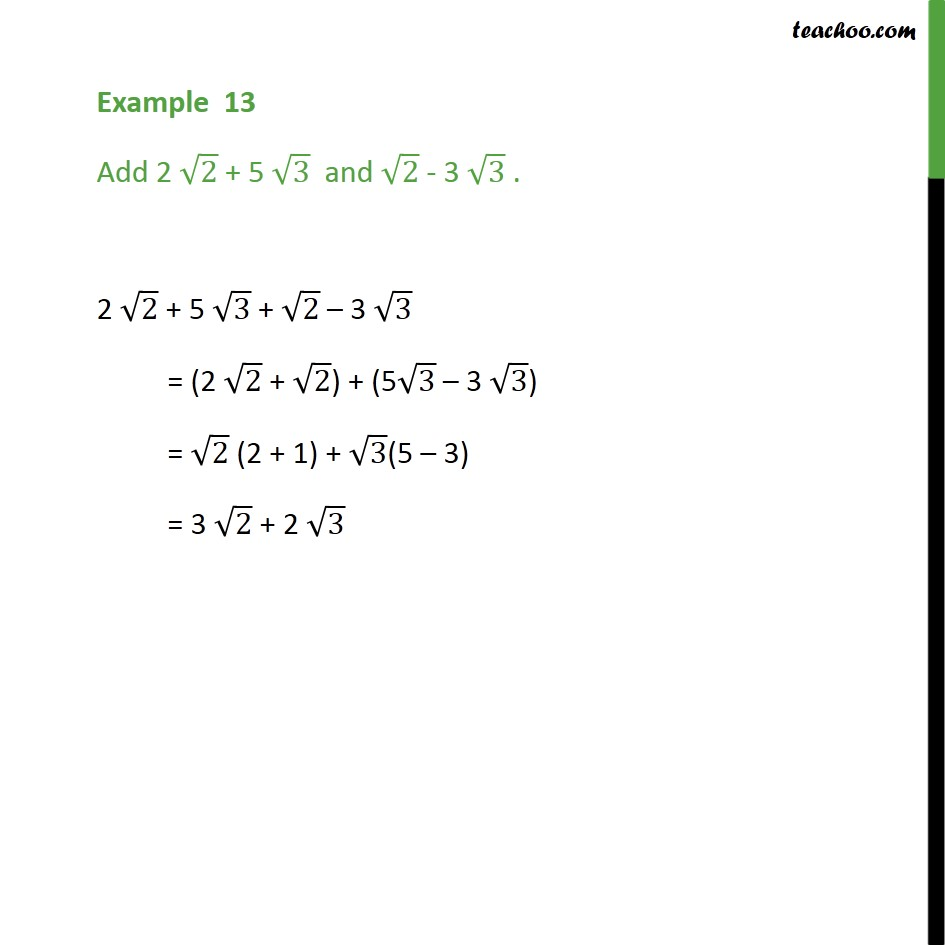1. Chapter 1 Class 9 Number Systems (Term 1)
2. Concept wise
3. Simplifying real numbers

Transcript

Example 13 Add 2 √2 + 5 √3 and √2 - 3 √3 . Here, both numbers have root in it So, we combine the numbers with the same root Combining 2 √2 & √2 and 5√3 & 3 √3, and then solving 2 √2 + 5 √3 + √2 – 3 √3 = (2 √2 + √2) + (5√3 – 3 √3) = √2 (2 + 1) + √3(5 – 3) = 3 √2 + 2 √3

Simplifying real numbers